# AP Statistics : How to find confidence intervals for the slope of a least-squares regression line

## Example Questions

### Example Question #1 : How To Find Confidence Intervals For The Slope Of A Least Squares Regression Line

You estimate a regression model with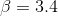and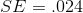, where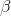is the beta coefficient and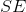is the standard error.  Construct 95% confidence intervals for.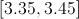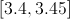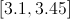To construct 95% confidence intervals for, we simply take the coefficient and add/subtract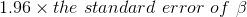. This is becauseis assumed to follow a symmetrical distribution (the normal), and 95% of the values in the sampling distribution are contained within 1.96 standard errors of.